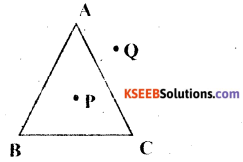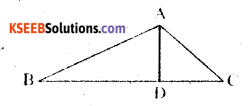# KSEEB Solutions for Class 6 Maths Chapter 4 Basic Geometrical Ideas Ex 4.4

Students can Download Chapter 4 Basic Geometrical Ideas Ex 4.4 Questions and Answers, Notes Pdf, KSEEB Solutions for Class 6 Maths helps you to revise the complete Karnataka State Board Syllabus and score more marks in your examinations.

## Karnataka State Syllabus Class 6 Maths Chapter 4 Basic Geometrical Ideas Ex 4.4

Question 1.
Draw a rough sketch of a triangle ABC. Mark a point Pin its interior and a Point Q in its exterior. Is the point A in its exterior or in its interior?
Solution:Point A lines on the given ∆ ABCQuestion 2.Solution:
a) Identify three triangles in the figure
∆ABC, ∆ACD, ∆ABD

b) Write the names of seven angles$$\overline{\mathrm{AB}}$$, $$\overline{\mathrm{BC}}$$, $$\overline{\mathrm{CA}}$$, $$\overline{\mathrm{AD}}$$, $$\overline{\mathrm{BD}}$$, $$\overline{\mathrm{CD}}$$